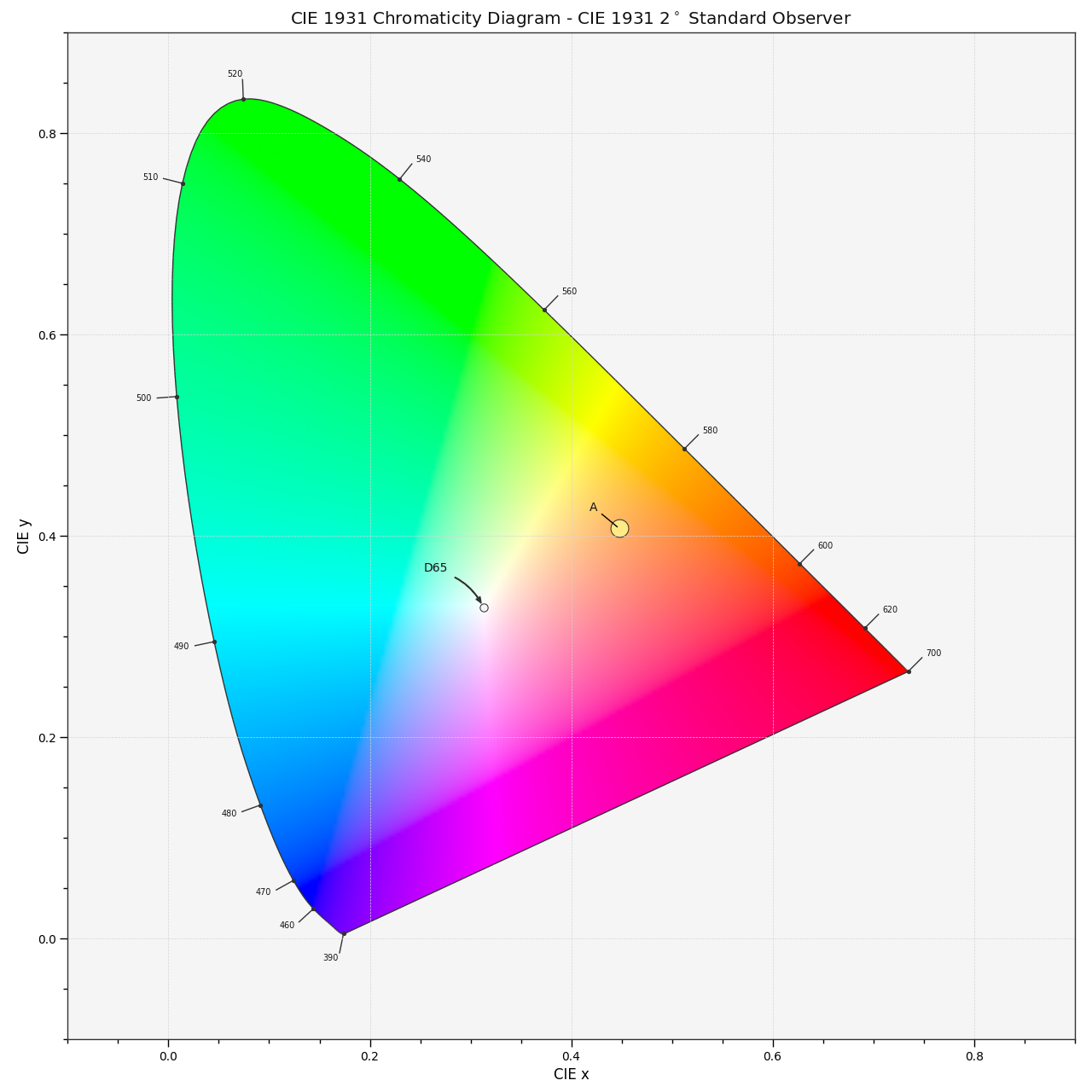# colour.plotting.diagrams.plot_sds_in_chromaticity_diagram#

colour.plotting.diagrams.plot_sds_in_chromaticity_diagram(sds: , cmfs: = 'CIE 1931 2 Degree Standard Observer', chromaticity_diagram_callable: Callable = plot_chromaticity_diagram, method: Union[Literal['CIE 1931', 'CIE 1960 UCS', 'CIE 1976 UCS'], str] = 'CIE 1931', annotate_kwargs: Optional[Union[Dict, List[Dict]]] = None, plot_kwargs: Optional[Union[Dict, List[Dict]]] = None, **kwargs: Any) [source]#

Plot given spectral distribution chromaticity coordinates into the Chromaticity Diagram using given method.

Parameters:
• sds (Union[Sequence[Union[SpectralDistribution, MultiSpectralDistributions]], MultiSpectralDistributions]) – Spectral distributions or multi-spectral distributions to plot. sds can be a single `colour.MultiSpectralDistributions` class instance, a list of `colour.MultiSpectralDistributions` class instances or a list of `colour.SpectralDistribution` class instances.

• cmfs (Union[MultiSpectralDistributions, str, Sequence[Union[MultiSpectralDistributions, str]]]) – Standard observer colour matching functions used for computing the spectral locus boundaries. `cmfs` can be of any type or form supported by the `colour.plotting.filter_cmfs()` definition.

• chromaticity_diagram_callable (Callable) – Callable responsible for drawing the Chromaticity Diagram.

• method (Union[Literal['CIE 1931', 'CIE 1960 UCS', 'CIE 1976 UCS'], str]) – Chromaticity Diagram method.

• annotate_kwargs (Optional[Union[Dict, List[Dict]]]) –

Keyword arguments for the `matplotlib.pyplot.annotate()` definition, used to annotate the resulting chromaticity coordinates with their respective spectral distribution names. `annotate_kwargs` can be either a single dictionary applied to all the arrows with same settings or a sequence of dictionaries with different settings for each spectral distribution. The following special keyword arguments can also be used:

• `annotate` : Whether to annotate the spectral distributions.

• plot_kwargs (Optional[Union[Dict, List[Dict]]]) –

Keyword arguments for the `matplotlib.pyplot.plot()` definition, used to control the style of the plotted spectral distributions. plot_kwargs` can be either a single dictionary applied to all the plotted spectral distributions with the same settings or a sequence of dictionaries with different settings for each plotted spectral distributions. The following special keyword arguments can also be used:

• `illuminant` : The illuminant used to compute the spectral distributions colours. The default is the illuminant associated with the whitepoint of the default plotting colourspace. `illuminant` can be of any type or form supported by the `colour.plotting.filter_cmfs()` definition.

• `cmfs` : The standard observer colour matching functions used for computing the spectral distributions colours. `cmfs` can be of any type or form supported by the `colour.plotting.filter_cmfs()` definition.

• `normalise_sd_colours` : Whether to normalise the computed spectral distributions colours. The default is True.

• `use_sd_colours` : Whether to use the computed spectral distributions colours under the plotting colourspace illuminant. Alternatively, it is possible to use the `matplotlib.pyplot.plot()` definition `color` argument with pre-computed values. The default is True.

• kwargs (Any) – {`colour.plotting.artist()`, `colour.plotting.diagrams.plot_chromaticity_diagram()`, `colour.plotting.render()`}, See the documentation of the previously listed definitions.

Returns:

Current figure and axes.

Return type:

`tuple`

Examples

```>>> A = SDS_ILLUMINANTS['A']
>>> D65 = SDS_ILLUMINANTS['D65']
>>> annotate_kwargs = [
...     {'xytext': (-25, 15), 'arrowprops':{'arrowstyle':'-'}},
...     {}
... ]
>>> plot_kwargs = [
...     {
...         'illuminant': SDS_ILLUMINANTS['E'],
...         'markersize' : 15,
...         'normalise_sd_colours': True,
...         'use_sd_colours': True
...     },
...     {'illuminant': SDS_ILLUMINANTS['E']},
... ]
>>> plot_sds_in_chromaticity_diagram(
...     [A, D65], annotate_kwargs=annotate_kwargs, plot_kwargs=plot_kwargs)
...
(<Figure size ... with 1 Axes>, <...AxesSubplot...>)
```